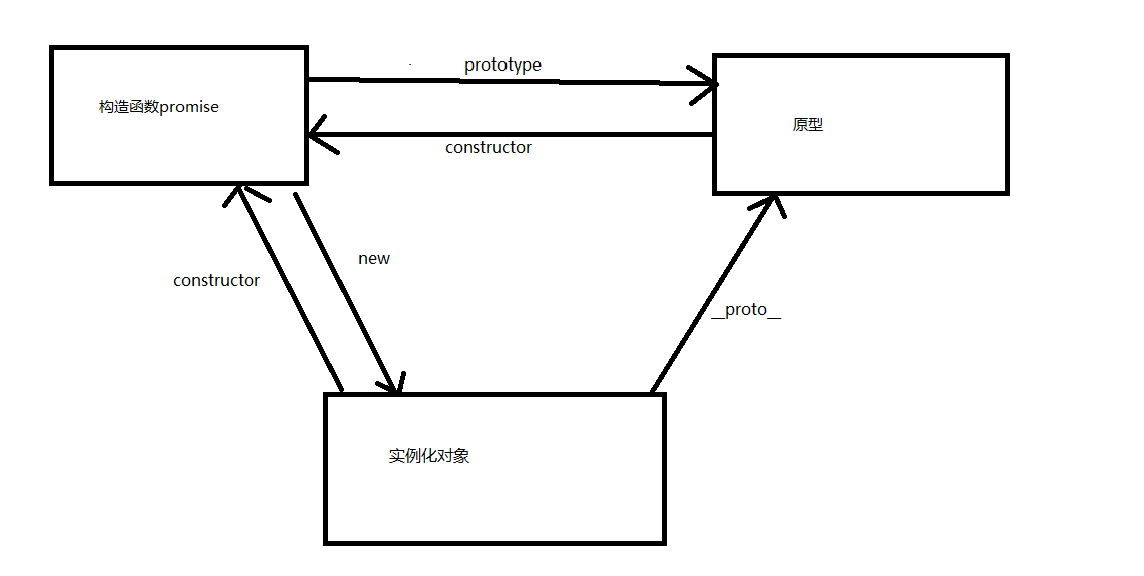## 深拷贝+浅拷贝+数据类型判断

2021/1/13 20:39:18 文章标签: 测试文章如有侵权请发送至邮箱809451989@qq.com投诉后文章立即删除

# 深拷贝浅拷贝的原理

• ## 浅拷贝

• 浅拷贝就是对数据的复制
• ### 浅拷贝的使用方法

``````	// 浅拷贝的方法
let arr = [1,2,3,4];
let newArr = arr;
``````
• 浅拷贝的缺点
当你创建一个新的变量newArr直接复制已有变量的数据时，你改变原变量arr数据，新的变量newArr数据也会发生改变，最终两个的变量值是一样的
``````   // 浅拷贝的方法
let arr = [1,2,3,4];
let newArr = arr;
arr = 999;
console.log(arr,newArr) // 结果[1,2,3,999] [1,2,3,999];
``````
``````	let arr = [1,2,3,4];
let newArr = [...arr];
arr = 999;
// 改变原数据的值，不会影响深拷贝后的数据
console.log(arr,newArr) // 结果[1,2,3,999] [1,2,3,4];
``````
• 缺点：不会改变深层次数据的指针，深层次数据的指针还是指向原数据，否则改变原数据的深层次深拷贝后的数据的深层次也会发生改变
``````	let arr = [1,2,3,4,{name:'李四'}];
let newArr = [...arr];
arr[arr.length - 1].name = '张三';
console.log(arr,newArr) // 结果[1,2,3,4,{name:'张三'}],[1,2,3,4,{name:'张三'}]
``````
• #### json转换的方法

• son转换将数据转换成字符串，在转换为原数据类型，复制给新变量
``````	let arr = [1,2,3,4,{name:'李四'}];
let newArr = json.parse(json.stringify(arr))
arr[arr.length - 1].name = '张三';
console.log(arr,newArr) // 结果[1,2,3,4,{name:'张三'}],[1,2,3,4,{name:'李四'}]
``````
• #### Object.assign()方法

• 他有两个参数，第一个是要合并的类型，第二个是合并的目标，改变原数据不会影响深拷贝后的数据
``````	let arr = [1,2,3,4,{name:'李四'}];
let newArr = Object.assign([],arr)
arr = 999
console.log(arr,newArr);
``````
• 缺点：不会改变深层次数据的指针，深层次数据的指针还是指向原数据，否则改变原数据的深层次深拷贝后的数据的深层次也会发生改变
``````	let arr = [1,2,3,4,{name:'李四'}];
let newArr = Object.assign([],arr)
arr[arr.length - 1].name = '张三';
console.log(arr,newArr) // 结果[1,2,3,4,{name:'张三'}],[1,2,3,4,{name:'张三'}]
``````
• #### 递归方法

``````	var obj = { // 定义要进行深复制的对象
name: "张三",
age: 14,
tel: 110,
info: {
isSingle: true
}
}
// 定义深复制的函数
function deepClone(params) {
// 创建一个接受递归后数据的变量
var obj = {}
// 如果形参的数据不是Object的话return
if(!(params instanceof Object)) return
// 循环形参的数据
for(let key in params) {
// 判断循环出的数据是否为Object
if(params[key] instanceof Object) {
// 如果是Object拿之前的创建的变量接收一个函数的自我调用，将Object以实参传递给当前函数
obj[key] = deepClone(params[key])
// 循环出的数据不为Object
} else {
// 将数据直接赋值给生成的变量
obj[key] = params[key]
}
}
// 将接收的数据return出去
return obj
}
// 验证结论
var obj2 = deepClone(obj)
obj2.name = '李四'
obj2.info.isSingle = false
console.log(obj, obj2)
``````

# 原型

• 以图参考# 数据类型

• #### 判断数据类型的四种方法

• ##### typeOf：主要判断基本数据类型
``````	let arr = 1;
console.log(typeOf(arr)) // 结果Number
let arr2 = 'abc';
console.log(typeOf(arr2)) // 结果String
``````
• 缺点：当数据为引用数据类型时，判断出结果就是字符串object
``````	let arr = [1,231,4];
console.log(typeOf(arr)) // 结果object
``````
• ##### instanceOf：主要用于判断引用数据类型
``````	let arr = [1,231,4];
console.log(arr instanceof Array); // 结果为true
``````
• 缺点：当数据为基本数据类型时，判断出结果就是false
``````		let num = 1;
let num2 = '1';
console.log(num instanceof Number); // 结果为false
console.log(num2 instanceof String); // 结果为false
``````
• constructor：对引用数据类型和基本数据类型都有很好的判断，但是如果生成一个函数，把他的原型指向数组后者对象，那么他的判断结果就不一定是函数了
• ##### Object.prototype.toString.call()：完美判断所有数据的类型，如果需要判断深层数据时，需要使用递归的方式
``````	let arr = [1,2,3,4,{name:'李四'}];
let num = 1;
let str = '1';
let obj = {name:'张三'};
console.log(Object.prototype.toString.call(arr)); // 结果为 [object Array]
console.log(Object.prototype.toString.call(num)); // 结果为 [object Number]
console.log(Object.prototype.toString.call(str)); // 结果为 [object String]
console.log(Object.prototype.toString.call(obj)); // 结果为 [object Object]
``````

暂无相关的数据...